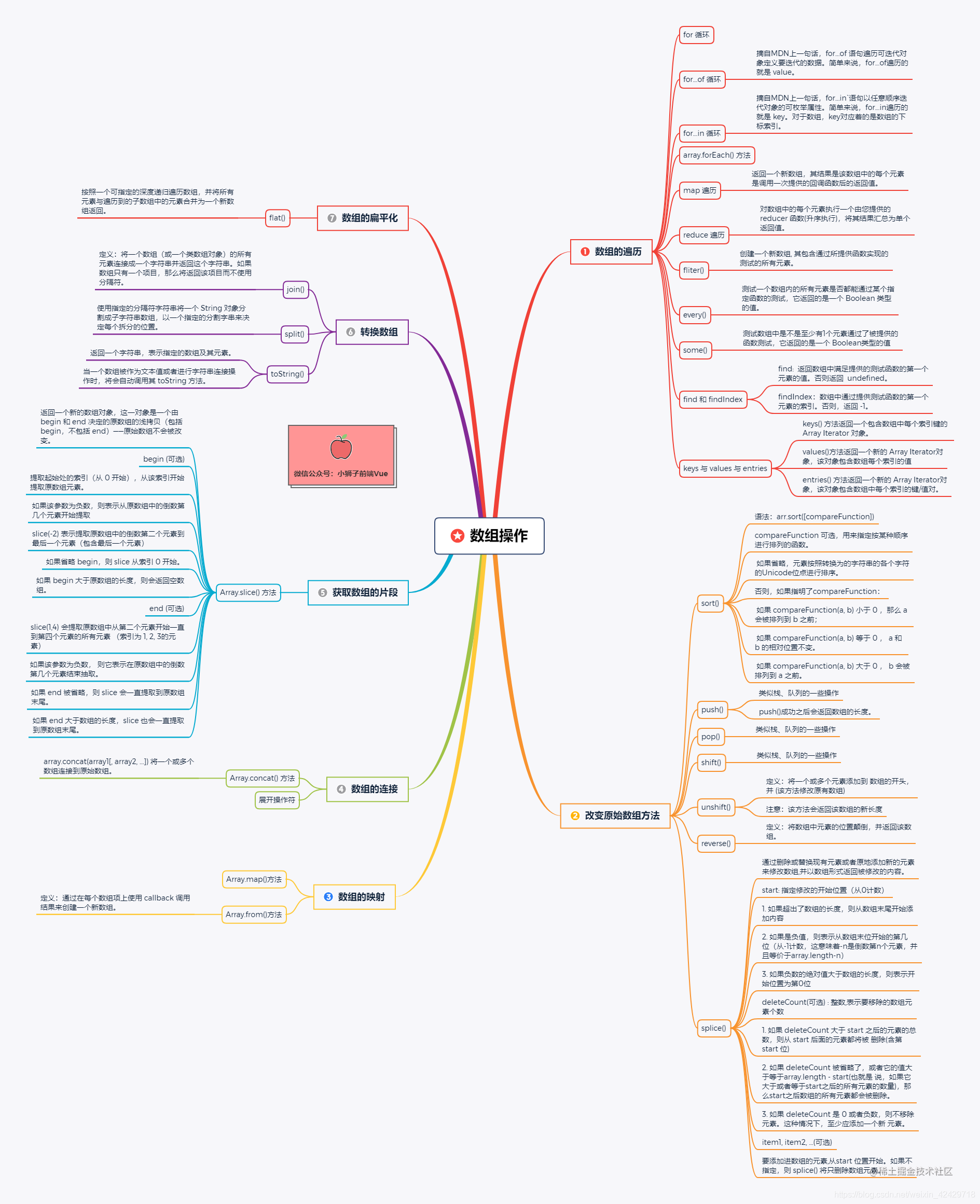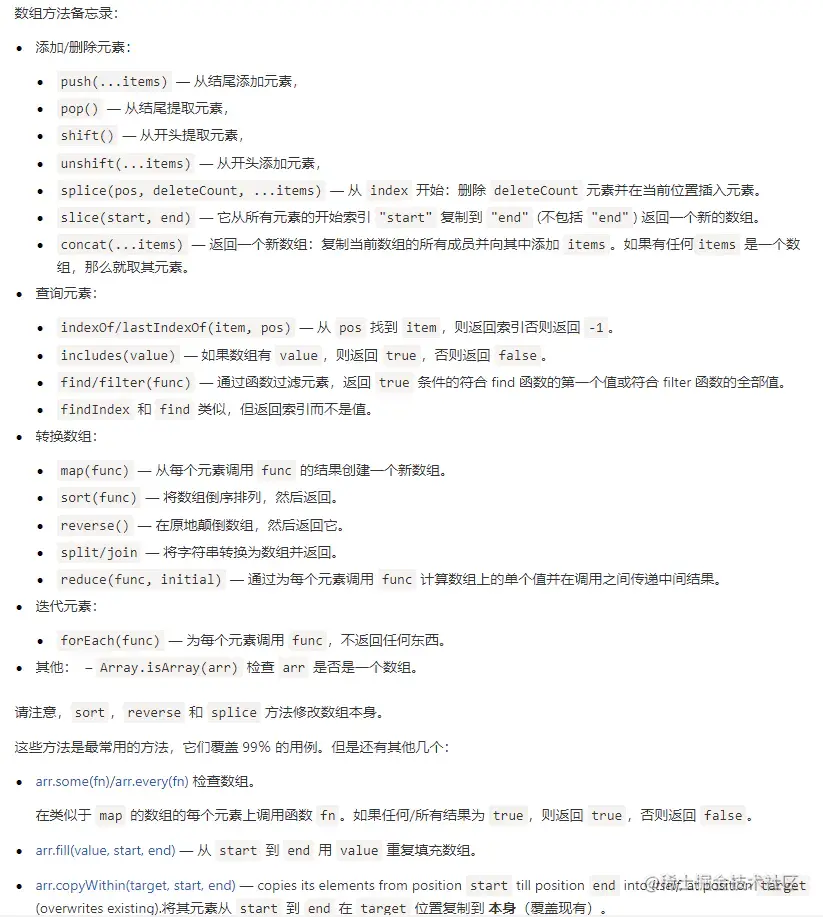# 「数组方法」写给女友的一系列 JS 数组操作（建议收藏 | 内附思维导图）## 前言（手机端可能看不清）获取高清PDF，请在微信公众号【小狮子前端Vue】回复【数组方法】

## 数组的遍历

### for 循环

``````let arr = [1, 2, 3, 4, 5];
for (let i = 0; i < arr.length; i++) {
console.log(arr[i]);
}
// 1
// 2
// 3
// 4
// 5

### for..of 循环

``````let arr = ['Chocolate', 'zhlll', 'lionkk'];

for (let val of arr) {
console.log(val);
}
// Chocolate
// zhlll
// lionkk

### for...in 循环

``````let arr = ['Chocolate', 'zhlll', 'lionkk'];

for (let key in arr) {
console.log(key);
}
// 0
// 1
// 2

### array.forEach() 方法

``````array.forEach(callback(currentValue, index, arr), thisArg)

callback:为数组中每个元素执行的函数，该函数接收一至三个参数

currentValue 数组中正在处理的当前元素 index (可选) 数组中正在处理的当前元素的索引 arr (可选) forEach() 方法正在操作的数组 thisArg 可选参数,当执行回调函数callback,用作this值

``````let arr = ['Chocolate', 'zhlll', 'lionkk'];

arr.forEach(function (cur, index, arr) {
console.log(cur, index, arr);
})

// Chocolate 0 [ 'Chocolate', 'zhlll', 'lionkk' ]
// zhlll 1 [ 'Chocolate', 'zhlll', 'lionkk' ]
// lionkk 2 [ 'Chocolate', 'zhlll', 'lionkk' ]

``````let arr = ['Chocolate', 'zhlll', 'lionkk'];

arr.forEach((cur) => {
console.log(cur);
})
// Chocolate
// zhlll
// lionkk

``````function Foo() {
this.sum = 0;
this.cnt = 0;
}
// 在原型上添加一个名为 doSth 方法
Foo.prototype.doSth = function (arr) {
arr.forEach(function (cur) {
this.sum += cur;
this.cnt++;
}, this) // this 指向实例对象
}

let foo = new Foo();
let arr = [1, 2, 3];
foo.doSth(arr);

console.log(foo.sum, foo.cnt);
// 6 3
// 解释： 6 === (1+2+3)  3 === (1+1+1)

### map 遍历

``````let newArray = array.map(function(currentValue, index, arr), thisArg)

callback:为数组中每个元素执行的函数，该函数接收一至三个参数

currentValue 数组中正在处理的当前元素 index (可选) 数组中正在处理的当前元素的索引 arr (可选) map() 方法正在操作的数组 thisArg 可选参数,当执行回调函数callback,用作this值

``````let arr = ['Chocolate', 'zhlll', 'lionkk'];

let newArr = arr.map(function (cur, index, arr) {
console.log(cur, index, arr);
return cur + index;
})
// Chocolate 0 [ 'Chocolate', 'zhlll', 'lionkk' ]
// zhlll 1 [ 'Chocolate', 'zhlll', 'lionkk' ]
// lionkk 2 [ 'Chocolate', 'zhlll', 'lionkk' ]

console.log(newArr)
// [ 'Chocolate0', 'zhlll1', 'lionkk2' ]

``````function Foo() {
this.sum = 0;
this.cnt = 0;
}
// 在原型上添加一个名为 doSth 方法
Foo.prototype.doSth = function (arr) {
let newArr = arr.map(function (cur) {
this.sum += cur;
this.cnt++;
return cur + 10;
}, this) // this 指向实例对象
return newArr;
}

let foo = new Foo();
let arr = [1, 2, 3];

console.log(foo.doSth(arr)); // [ 11, 12, 13 ]
console.log(foo.sum);// 6
console.log(foo.cnt);// 3

``````let arr = [1, 4, 9, 16];
let res = arr.map(Math.sqrt); // 传入Math中sqrt得到数组中每个元素的平方根
console.log(res); // [ 1, 2, 3, 4 ]

• `map` 不修改调用它的原数组本身（当然可以在 `callback` 执行时改变原数组）

• `this` 的值最终相对于 `callback` 函数的可观察性是依据this规则，也就是 `this` 指向问题
• `map` 会返回一个新数组

### reduce 遍历

``````let res= array.reduce(callback(accumulator, currentValue, currentIndex, array), initialValue)

callback:为数组中每个元素执行的函数，该函数接收一至4个参数

accumulator 累计器 currentValue 当前值 currentIndex 当前索引 array 数组 initialValue 作为第一次调用 callback函数时的第一个参数的值。 如果没有提供初始值，则将使用数组中的第一个元素。 在没有初始值的空数组上调用 reduce 将报错。

``````let arr = [3, 5, 7, 1, 2];
let res = arr.reduce(function (acc, cur, index, arr) {
console.log(acc, cur, index, arr);
return acc + cur;
}, 0)
// 0 3 0 [ 3, 5, 7, 1, 2 ]
// 3 5 1 [ 3, 5, 7, 1, 2 ]
// 8 7 2 [ 3, 5, 7, 1, 2 ]
// 15 1 3 [ 3, 5, 7, 1, 2 ]
// 16 2 4 [ 3, 5, 7, 1, 2 ]
console.log(res);
// 18

``````let arr = [1, 2, 3, 4, 5];
let res = arr.reduce((acc, cur) => {
return acc + cur;
}, 0)
console.log(res);// 15

``````[1, 2, 3, 4].reduce((x, y) => console.log(x, y));

• A: `1` `2` and `3` `3` and `6` `4`
• B: `1` `2` and `2` `3` and `3` `4`
• C: `1` `undefined` and `2` `undefined` and `3` `undefined` and `4` `undefined`
• D: `1` `2` and `undefined` `3` and `undefined` `4`

#### 答案: D

`reducer` 函数接收4个参数:

1. Accumulator (acc) (累计器)
2. Current Value (cur) (当前值)
3. Current Index (idx) (当前索引)
4. Source Array (src) (源数组)

`reducer` 函数的返回值将会分配给累计器，该返回值在数组的每个迭代中被记住，并最后成为最终的单个结果值。

`reducer` 函数还有一个可选参数`initialValue`, 该参数将作为第一次调用回调函数时的第一个参数的值。如果没有提供`initialValue`，则将使用数组中的第一个元素。

• 如果数组为空且没有提供 `initialValue`，会抛出`TypeError`
• 如果没有提供 `initialValue``reduce` 会从索引1的地方开始执行 `callback` 方法，跳过第一个索引。如果提供 `initialValue` ，从索引0开始。
• `acc`为传入函数的返回值，如果是 `console.log`，则返回默认值 `undefined`

### filter()

``````let newArray = array.filter(function(currentValue, index, arr), thisArg)

callback:为数组中每个元素执行的函数，该函数接收一至三个参数

currentValue 数组中正在处理的当前元素 index (可选) 数组中正在处理的当前元素的索引 arr (可选) filter() 方法正在操作的数组 thisArg（可选参数）,当执行回调函数callback,用作this值

``````let arr = [1, 2, 3, 4, 5];

let newArr = arr.filter(function (cur, index) {
console.log(cur, index);
return cur % 2 == 0;
})
// 1 0
// 2 1
// 3 2
// 4 3
// 5 4
console.log(newArr); // [ 2, 4 ]

### every()

``````array.every(function(currentValue, index, arr), thisArg)

callback:为数组中每个元素执行的函数，该函数接收一至三个参数

currentValue 数组中正在处理的当前元素 index (可选) 数组中正在处理的当前元素的索引 arr (可选) every() 方法正在操作的数组 thisArg 可选参数,当执行回调函数callback,用作this值

``````let res1 = [1, 2, 3, 4, 5].every(function (cur) {
return cur > 10;
})
console.log(res1); // false

let res2 = [1, 2, 3, 4, 5].every(function (cur) {
return cur >= 1;
})
console.log(res2); // true

### some()

``````array.some(function(currentValue, index, arr), thisArg)

callback:为数组中每个元素执行的函数，该函数接收一至三个参数

currentValue 数组中正在处理的当前元素 index (可选) 数组中正在处理的当前元素的索引 arr (可选) some() 方法正在操作的数组 thisArg （可选参数）,当执行回调函数callback,用作this值

``````let res1 = [1, 2, 3, 4, 5].some(function (cur) {
return cur > 10;
})
console.log(res1); // false

let res2 = [1, 2, 3, 4, 5].some(function (cur) {
return cur === 1;
})
console.log(res2); // true

### find 和 findIndex

`find`: 返回数组中满足提供的测试函数的第一个元素的值。否则返回 `undefined`

`findIndex`：数组中通过提供测试函数的第一个元素的索引。否则，返回 `-1`

``````let ele = array.find(function(elemnet, index, arr), thisArg)

let eleIndex = array.findIndex(function(elemnet, index, arr), thisArg)

callback:为数组中每个元素执行的函数，该函数接收一至三个参数

elemnet 数组中正在处理的当前元素 index (可选) 数组中正在处理的当前元素的索引 arr (可选) find方法正在操作的数组 thisArg 可选参数,当执行回调函数callback,用作this值

``````let res1 = [1, 2, 3, 4, 5].find(function (cur) {
return cur > 2;
})
console.log(res1); // 3

let res2 = [1, 2, 3, 4, 5].findIndex(function (cur) {
return cur > 2;
})
console.log(res2); // 2

### keys 与 values 与 entries

• `keys()` 方法返回一个包含数组中每个索引键的 Array Iterator 对象。
• `values()` 方法返回一个新的 `Array Iterator` 对象，该对象包含数组每个索引的值
• `entries()` 方法返回一个新的 `Array Iterator` 对象，该对象包含数组中每个索引的键/值对
``````arr.keys()
arr.values()
arr.entries()

``````let arr = ['Chocolate', 'zhlll', 'lionkk'];

let itKeys = arr.keys();
let itVals = arr.values();
let itEntries = arr.entries();

for (let it of itKeys) {
console.log(it);
}
// 0
// 1
// 2
for (let it of itVals) {
console.log(it);
}
// Chocolate
// zhlll
// lionkk
for (let it of itEntries) {
console.log(it);
}
// [ 0, 'Chocolate' ]
// [ 1, 'zhlll' ]
// [ 2, 'lionkk' ]

## 改变原始数组方法

### sort()

``````arr.sort([compareFunction])

compareFunction 可选，用来指定按某种顺序进行排列的函数。 如果省略，元素按照转换为的字符串的各个字符的Unicode位点进行排序。

``````let arr = [1, 10, 2, 5, 8, 3];

arr.sort(); // 默认
console.log(arr); // [ 1, 10, 2, 3, 5, 8 ]

arr.sort((a, b) => a - b); // 从小到大排序
console.log(arr); // [ 1, 2, 3, 5, 8, 10 ]

arr.sort((a, b) => b - a); // 从大到小排序
console.log(arr); // [ 10, 8, 5, 3, 2, 1 ]

### push()

``````let arr = [1,2];
let res = arr.push(100);
console.log(arr); // [ 1, 2, 100 ]
console.log(res); // 3

### pop()

``````let arr = [1, 2, 100];
let res = arr.pop();
console.log(arr); // [ 1, 2 ]
console.log(res); // 100

### shift()

``````let arr = [1, 2, 100];
let res = arr.shift();
console.log(arr); // [ 2, 100 ]
console.log(res); // 1

### unshift()

``````let arr = [1, 2, 100];
let res = arr.unshift(4, 5, 6);
console.log(arr); // [ 4, 5, 6, 1, 2, 100 ]
console.log(res); // 6

### reverse()

``````let arr = [1, 2, 3];
arr.reverse();
console.log(arr);// [ 3, 2, 1 ]

### splice()

``````array.splice(start,deleteCount,item1,.....,itemX)z

start: 指定修改的开始位置（从0计数）

``````	1. 如果超出了数组的长度，则从数组末尾开始添加内容
2. 如果是负值，则表示从数组末位开始的第几位（从-1计数，这意味着-n是倒数第n个元素，并且等价于array.length-n）
3. 如果负数的绝对值大于数组的长度，则表示开始位置为第0位

deleteCount(可选) : 整数,表示要移除的数组元素个数

``````	1. 如果 deleteCount 大于 start 之后的元素的总数，则从 start 后面的元素都将被			删除(含第 start 位)
2. 如果 deleteCount 被省略了，或者它的值大于等于array.length - start(也就是		   说，如果它大于或者等于start之后的所有元素的数量)，那么start之后数组的所有元素都会被删除。
3. 如果 deleteCount 是 0 或者负数，则不移除元素。这种情况下，至少应添加一个新		   元素。

item1, item2, ...(可选)

``````let arr = ['one', 'two', 'three'];

arr.splice(2, 0, 'Chocolate');
console.log(arr);// [ 'one', 'two', 'Chocolate', 'three' ]

``````let arr = ['one', 'two', 'three'];

arr.splice(2, 1, 'Chocolate');
console.log(arr);// [ 'one', 'two', 'Chocolate' ]

## 数组的映射

### Array.from()方法

`````` Array.from(Array,callback(currentValue, index, arr))

``````let arr = [1, 2, 3];

let newArr = Array.from(arr, function (cur) {
return cur + 10;
})
console.log(newArr);// [ 11, 12, 13 ]

## 数组的连接

### Array.concat() 方法

`array.concat(array1[, array2, ...])` 将一个或多个数组连接到原始数组。如下所示，连接两个数组：

``````let arrA = [1, 2, 3];
let arrB = [4, 5, 6];
let ans = arrA.concat(arrB);
console.log(ans);// [ 1, 2, 3, 4, 5, 6 ]

### 展开操作符

``````let arrA = [1, 2, 3];
let arrB = [4, 5, 6];
let ans = [...arrA, ...arrB];
console.log(ans);// [ 1, 2, 3, 4, 5, 6 ]

## 获取数组的片段

### Array.slice() 方法

``````arr.slice([begin[, end]])

begin (可选)

1. 提取起始处的索引（从 0 开始），从该索引开始提取原数组元素。
2. 如果该参数为负数，则表示从原数组中的倒数第几个元素开始提取
3. slice(-2) 表示提取原数组中的倒数第二个元素到最后一个元素（包含最后一个元素）
4. 如果省略 begin，则 slice 从索引 0 开始。
5. 如果 begin 大于原数组的长度，则会返回空数组。

end (可选)

1. slice(1,4) 会提取原数组中从第二个元素开始一直到第四个元素的所有元素 （索引为 1, 2, 3的元素）
2. 如果该参数为负数， 则它表示在原数组中的倒数第几个元素结束抽取。
3. 如果 end 被省略，则 slice 会一直提取到原数组末尾。
4. 如果 end 大于数组的长度，slice 也会一直提取到原数组末尾。
``````let fruits = ['Banana', 'Orange', 'Lemon', 'Apple', 'Mango'];
let res = fruits.slice(1, 3);
let res1 = fruits.slice(1);
let res2 = fruits.slice(-1);
let res3 = fruits.slice(0, -1);
console.log(res); // [ 'Orange', 'Lemon' ]
console.log(res1);// [ 'Orange', 'Lemon', 'Apple', 'Mango' ]
console.log(res2);// [ 'Mango' ]
console.log(res3);// [ 'Banana', 'Orange', 'Lemon', 'Apple' ]

## 转换数组

### join()

``````arr.join(separator)

``````let arr = ['one', 'two', 'three'];
let res = arr.join('^');
let res1 = arr.join('&');

console.log(res); // one^two^three
console.log(res1); // one&two&three

### split()

``````str.split([separator[, limit]])

``````const str = 'The best Chocolate';

const words = str.split(' ');
console.log(words); // [ 'The', 'best', 'Chocolate' ]
console.log(words); // Chocolate

### toString()

``````arr.toString()

``````let arr = ['one', 'two', 'three'];
console.log(arr.toString()); // one,two,three

## 数组的扁平化

### flat()

``````var newArray = arr.flat([depth])

depth 可选 指定要提取嵌套数组的结构深度，默认值为 1。 返回值 一个包含将数组与子数组中所有元素的新数组。

``````const arr1 = [0, 1, 2, [3, 4]];
console.log(arr1.flat()); // [ 0, 1, 2, 3, 4 ]

const arr2 = [0, 1, 2, [[[3, 4]]]];
console.log(arr2.flat(2)); // [ 0, 1, 2, [ 3, 4 ] ]

## 备忘录## 最后

leetcode-javascript：LeetCode 力扣的 JavaScript 解题仓库，前端刷题路线（思维导图）``````学如逆水行舟，不进则退